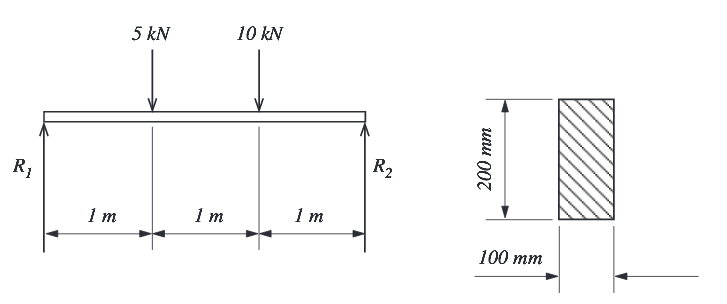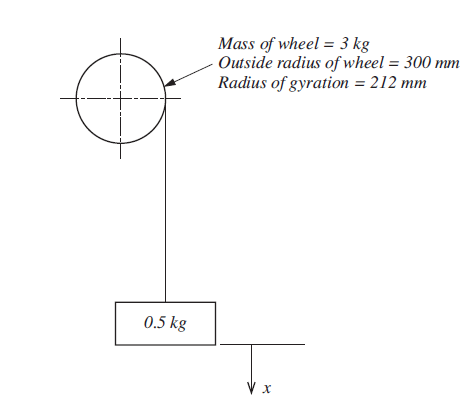Struggling with questions like these. Get One to One tuition with our trained HNC Engineering TutorsQ2 A beam of rectangular cross section 200 mm deep and 100 mm wide. If the beam is 3m long, simply supported at either end and carries point loads as shown in FIGURE 2
(a) Calculate the maximum bending moment
(b) Calculate the maximum stress in the beam3. A mass of 0.5 kg is suspended from a flywheel as shown in FIGURE 2. If the mass is released from rest and falls a distance of 0.5 m in 1.5 s, calculate:
(a) The linear acceleration of the mass.
(b) The angular acceleration of the wheel.Help is Just One Click Away